# The SURVEYLOGISTIC Procedure

### Linear Predictor, Predicted Probability, and Confidence Limits

Subsections:

This section describes how predicted probabilities and confidence limits are calculated by using the pseudo-estimates (MLEs) obtained from PROC SURVEYLOGISTIC. For a specific example, see the section Getting Started: SURVEYLOGISTIC Procedure. Predicted probabilities and confidence limits can be output to a data set with the OUTPUT statement.

#### Cumulative Response Models

For a row vector of explanatory variables, the linear predictor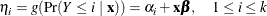is estimated by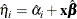where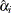and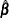are the MLEs of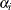and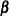. The estimated standard error of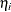is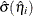, which can be computed as the square root of the quadratic form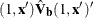, where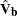is the estimated covariance matrix of the parameter estimates. The asymptotic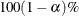confidence interval foris given by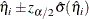where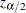is the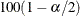percentile point of a standard normal distribution.

The predicted value and theconfidence limits for Pr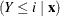are obtained by back-transforming the corresponding measures for the linear predictor.

Predicted ProbabilityConfidence Limits

LOGIT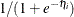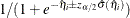PROBIT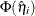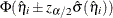CLOGLOG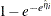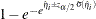#### Generalized Logit Model

For a vector of explanatory variables, let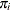denote the probability of obtaining the response value i: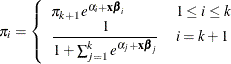By the delta method,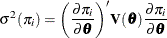A 100(1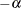)% confidence level foris given by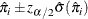where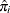is the estimated expected probability of response i and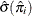is obtained by evaluating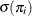at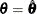.